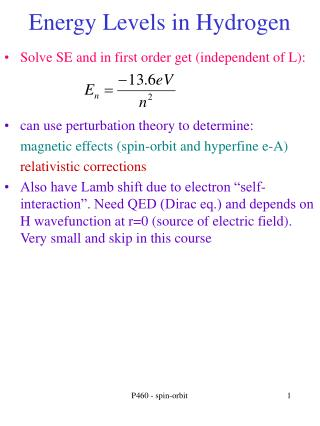DownloadDownload PresentationEnergy Levels in Hydrogen

# Energy Levels in Hydrogen

Télécharger la présentation## Energy Levels in Hydrogen

- - - - - - - - - - - - - - - - - - - - - - - - - - - E N D - - - - - - - - - - - - - - - - - - - - - - - - - - -
##### Presentation Transcript

1. Energy Levels in Hydrogen • Solve SE and in first order get (independent of L): • can use perturbation theory to determine: • magnetic effects (spin-orbit and hyperfine e-A) • relativistic corrections • Also have Lamb shift due to electron “self-interaction”. Need QED (Dirac eq.) and depends on H wavefunction at r=0 (source of electric field). Very small and skip in this course P460 - spin-orbit

2. Spin-Orbit Interactions • A non-zero orbital angular momentum L produces a magnetic field • electron sees it. Its magnetic moment interacts giving energy shift • in rest frame of electron, B field is (see book): • convert back to lab frame (Thomas precession due to non-inertial frame gives a factor of 2). Energy depends on spin-orbit coupling P460 - spin-orbit

3. Spin-Orbit: Quantum Numbers • The spin-orbit coupling (L*S) causes ml and ms to no longer be “good” quantum numbers • spin-orbit interactions changes energy. • In atomic physics, small perturbation, and can still use H spatial and spin wave function as very good starting point. Large effects in nuclear physics (and will see energy ordering very different due to couplings) P460 - spin-orbit

4. SL Expectation value • Determine expectation value of the spin-orbit interaction using perturbation theory. Assume J,L,S are all “good” quantum numbers (which isn’t true) • assume H wave function is ~eigenfunction of perturbed potential P460 - spin-orbit

5. SL Expectation value • To determine the energy shift, also need the expectation value of the radial terms using Laguerre polynomials • put all the terms together to get spin-orbit energy shift. =0 if l=0 J=3/2 j=1/2 L=1 P460 - spin-orbit

6. Spin Orbit energy shift • For 2P state. N=2, L=1, J= 3/2 or 1/2 • and so energy split between 2 levels is J=3/2 j=1/2 L=1 P460 - spin-orbit

7. Relativistic Effects • Solved using non-relativistic S.E. can treat relativistic term (Krel) as a perturbation • <V> can use virial theorom P460 - spin-orbit

8. Relativistic+spin-orbit Effects • by integrating over the radial wave function • combine spin-orbit and relativistic corrections • energy levels depend on only n+j (!). Dirac equation gives directly (not as perturbation) P460 - spin-orbit

9. Energy Levels in Hydrogen # states N=3 • Degeneracy = 2j+1 • spectroscopic notation: nLj with L=0 S=state, L=1 P-state, L=2 D-state E N=2 N=1 P460 - spin-orbit

10. Hyperfine Splitting • Many nuclei also have spin • p,n have S=1/2. Made from 3 S=1/2 quarks (plus additional quarks and antiquarks and gluons). G-factors are 5.58 and -3.8 from this (-2 for electron). • Nuclear g-factors/magnetic moments complicated. Usually just use experimental number • for Hydrogen. Let I be the nuclear spin (1/2) • have added terms to energy. For S-states, L=0 and can ignore that term P460 - spin-orbit

11. Hyperfine Splitting • Electron spin couples to nuclear spin • so energy difference between spins opposite and aligned. Gives 21 cm line for hydrogen (and is basis of NMR/MRI) P460 - spin-orbit